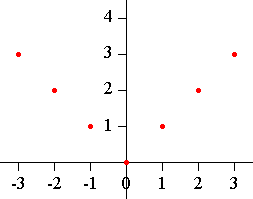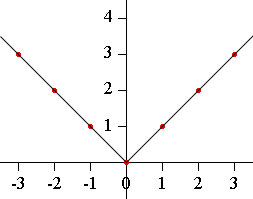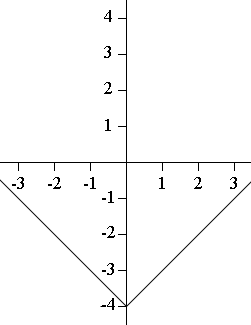SEARCH HOMEMath Central Quandaries & QueriesQuestion from tiana, a student: how do you find where to plot Y in a problem that states y=|x| -4?We have two responses for you

Tiana,

I would first plot some points to sketch the graph of y = |x|.

Make a table

x y = |x|
0 0
1 1
2 2
3 3
-1 1
-2 2
-3 3

plot the pointssketch the graph.y = |x|

For the function y = |x| - 4 each y-value is 4 less than the corresponding y-value for y = |x|.y = |x|y = |x| - 4

I hope this helps,
Penny

Two possibilities:

(1) The brute force method, on which everybody has to fall back sometimes, is to choose a lot of values of x, compute |x| and then |x| - 4 for each of them, and plot the point. Then draw a curve through the points. It helps to know that the absolute value function, unlike most others, really does yield a graph with a "kink" in it; it is thus an exception to the usual situation when a smooth curve is appropriate.

(2) By transformation: if you know what the graph of y=|x| looks like (and it is useful to know), you can lower it 4 units to get the graph you want. You can use the same technique to plot things like y = sin(x) +3, y = x^2 - 1, etc.

Good Hunting!
-RDMath Central is supported by the University of Regina and The Pacific Institute for the Mathematical Sciences.